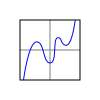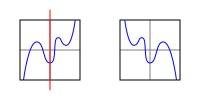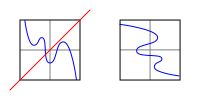# Transformation of a graph (function) - rotation 90 counter clockwise

#### Maths_Mike

##### New member
I know that to transform a graph 90 degrees counter clockwise you need to replace x with y and replace y with -x in the function.

Can anyone please explain why this is the case because if you apply this rule to a coordinate point it appears to rotate it 90 degrees clockwise. i.e (3,1) would go to (1,-3)

#### Mr. Bland

##### Junior Member
Imagine you have some coordinate axes printed on a square transparent sheet. There is a graph of some sort plotted on it as well.You manipulate the orientation of the sheet in order to transform the graph.

You begin by flipping the sheet over horizontally: everything on the left side of the graph moves to the right and everything on the right moves to the left. The vertical coordinates for all points on the graph remain unchanged. The new graph is effectively $$\displaystyle x' = -x, y' = y$$.Next you flip the sheet over a second time by holding the bottom-left and top-right corners, such that they remain where they are but the top-left and bottom-right corners exchange positions. This effectively exchanges the X and Y coordinates for each point on the graph: $$\displaystyle x' = y, y' = x$$. However, since the sheet had already been flipped from the previous step, the final transformation becomes $$\displaystyle x' = y, y' = -x$$.When you look at the graph, you see that it is rotated 90 degrees clockwise from where it started.

#### Maths_Mike

##### New member
so in your above example the 90 clockwise rotation is equivalent to replacing x by -y and y by x because x = -y' and y = x'

so for a 90 counter clockwise rotation I would be right as x' = -y and y' = x ???

Still a bit confused !

#### Jomo

##### Elite Member
This is not a proof but it makes the point, I hope.
Consider the point (1,10). So x is a small number and y is a large number. Now rotate this point 90 degrees clockwise. Note that (0, 10) will go to (10, 0) so (1,10) will go below the x-axis. Now the x value will be positive and long, so x will be 10. The y value will be small and negative, so y will be -1. That is (1, 10) goes to (10,-1). That is (x,y) goes to (y,-x)

I hope that helps a bit.

#### Maths_Mike

##### New member
Yes but the point is if you take a function y=f(x) and do the same thing I.e. replace x with y and y with -x then the transformation is 90 anti clockwise. The other way and that's what I am struggling to explain.

•hoosie

#### Jomo

##### Elite Member
Which other way?

What you wrote is wrong. If (x,y) becomes (y,-x) (ie x is replaced with y and y is replaced with -x) it is NOT a 90 degree rotation anticlockwise. It is a 90 degree rotation clockwise.

If by the other way, you mean 90 degree anti clockwise, then place a point (x,y) in the first quadrant. Let the position of the x-value be smaller in comparison to the y-value. Now rotate this point 90 degree anti clockwise. Figure out what this point will be. Hint: The point will be (x,y), (x,-y), (-x,y), (-x,-y), (y,x), (y,-x), (-y,x) or (-y,-x).

Claimer: This method is not a proof but it does get the job done.

#### Maths_Mike

##### New member
Consider the parabola y= x
Replace x with y and y with -x
(-x)=y
Y = x
Which if I am not mistaken means the graph has rotated anti clockwise.

#### Maths_Mike

##### New member
Ysquared = x

Going to y = x squared

#### Jomo

##### Elite Member
Consider the parabola y= x
Replace x with y and y with -x
(-x)=y
Y = x
Which if I am not mistaken means the graph has rotated anti clockwise.
y=x is not a parabola but rather a line.

You picked the only line that if rotated 90 degrees clockwise or 90 degrees counter clockwise gives the same line.

#### Maths_Mike

##### New member
Clearly not as 90 clockwise gives y = - x squared

#### Maths_Mike

##### New member
Sorry I typed incorrectly.
Ysquared = x which is a parabola
Following replace x with y and y with -x gives ysquared = y

It seems your coordinate argument is correct and that is how I tried to explain it. But it's the opposite rule when you transform a function which is the point of my thread

#### Maths_Mike

##### New member
Following replacement you get xsquared = y.

I need to turn off auto typing

Last edited:

Thank you.

#### hoosie

##### Junior Member
Which other way?

What you wrote is wrong. If (x,y) becomes (y,-x) (ie x is replaced with y and y is replaced with -x) it is NOT a 90 degree rotation anticlockwise. It is a 90 degree rotation clockwise.

If by the other way, you mean 90 degree anti clockwise, then place a point (x,y) in the first quadrant. Let the position of the x-value be smaller in comparison to the y-value. Now rotate this point 90 degree anti clockwise. Figure out what this point will be. Hint: The point will be (x,y), (x,-y), (-x,y), (-x,-y), (y,x), (y,-x), (-y,x) or (-y,-x).

Claimer: This method is not a proof but it does get the job done.
The OP understands that if a point (x,y) becomes (y,-x) it is being rotated 90 deg clockwise about the origin. To use your example (1,10) becomes (10,-1).

The student said “if you take a function y=f(x) and do the same thing i.e replace x with y and y with -x then the transformation is 90 deg anticlockwise.”

I was wondering why you said the student was wrong?

I can only assume you were not aware he was referring to the rule of a function when he said replacing x with y and y with -x produces a 90 deg anticlockwise rotation. With regard to a function this is a correct statement. With regard to a point the rotation is 90 deg clockwise as you indicated in your post.

Consider y = x² If we replace x with y and y with -x in the rule we get:

-x = y² i.e x = - y² This represents a sideways parabola which is concave to the left (a rotation of 90 deg anticlockwise). The opposite is the case when we replace x with y and y with -x in the coordinates of a point - the result is a 90 deg clockwise rotation as shown by your example.

•Maths_Mike

#### Maths_Mike

##### New member
The OP understands that if a point (x,y) becomes (y,-x) it is being rotated 90 deg clockwise about the origin. To use your example (1,10) becomes (10,-1).

The student said “if you take a function y=f(x) and do the same thing i.e replace x with y and y with -x then the transformation is 90 deg anticlockwise.”

I was wondering why you said the student was wrong?

I can only assume you were not aware he was referring to the rule of a function when he said replacing x with y and y with -x produces a 90 deg anticlockwise rotation. With regard to a function this is a correct statement. With regard to a point the rotation is 90 deg clockwise as you indicated in your post.

Consider y = x² If we replace x with y and y with -x in the rule we get:

-x = y² i.e x = - y² This represents a sideways parabola which is concave to the left (a rotation of 90 deg anticlockwise). The opposite is the case when we replace x with y and y with -x in the coordinates of a point - the result is a 90 deg clockwise rotation as shown by your example.
It was the other way round.I gave the function rule. The student referring to coordinates thought I was wrong. I knew I wasn't but couldn't explain why.
Thanks to all for help.

#### hoosie

##### Junior Member
It was the other way round.I gave the function rule. The student referring to coordinates thought I was wrong. I knew I wasn't but couldn't explain why.
Thanks to all for help.
Sorry Mike I should have said OP and not student in my last post.

•Maths_Mike

#### pka

##### Elite Member
I know that to transform a graph 90 degrees counter clockwise you need to replace x with y and replace y with -x in the function.
Can anyone please explain why this is the case because if you apply this rule to a coordinate point it appears to rotate it 90 degrees clockwise. i.e (3,1) would go to (1,-3)
Because this was posted in the intermediate/Advanced Algebra forum , I am surprised no one has responded with a matrix solution.
If $${A_\phi } =\displaystyle{ \left( {\begin{array}{*{20}{c}} {\cos (\phi )}&{ - \sin (\phi )} \\ {\sin (\phi )}&{\cos (\phi )} \end{array}} \right)}$$ [LaTeX error]
That matrix rotates points clockwise through an angle of $$\phi$$. To do it counterclockwise simply use $$-\phi$$.
Because $$\cos(-90^o)=0~\&~\sin(-90^o)=-1$$ we get the matrix $$\displaystyle \left( {\begin{array}{*{20}{c}} 0&1 \\ { {- 1}}&0 \end{array}} \right)$$ [that is $$-1$$ another LaTeX error]
The LaTex prevents witting this correctly but that matrix acts on $$(x,y)\to(y,-x)$$

#### Jomo

##### Elite Member
Because this was posted in the intermediate/Advanced Algebra forum , I am surprised no one has responded with a matrix solution.
If $${A_\phi } =\displaystyle{ \left( {\begin{array}{*{20}{c}} {\cos (\phi )}&{ - \sin (\phi )} \\ {\sin (\phi )}&{\cos (\phi )} \end{array}} \right)}$$ [LaTeX error]
That matrix rotates points clockwise through an angle of $$\phi$$. To do it counterclockwise simply use $$-\phi$$.
Because $$\cos(-90^o)=0~\&~\sin(-90^o)=-1$$ we get the matrix $$\displaystyle \left( {\begin{array}{*{20}{c}} 0&1 \\ { {- 1}}&0 \end{array}} \right)$$ [that is $$-1$$ another LaTeX error]
The LaTex prevents witting this correctly but that matrix acts on $$(x,y)\to(y,-x)$$
I thought of that but suspected that the student was in intermediate algebra and not advanced algebra.